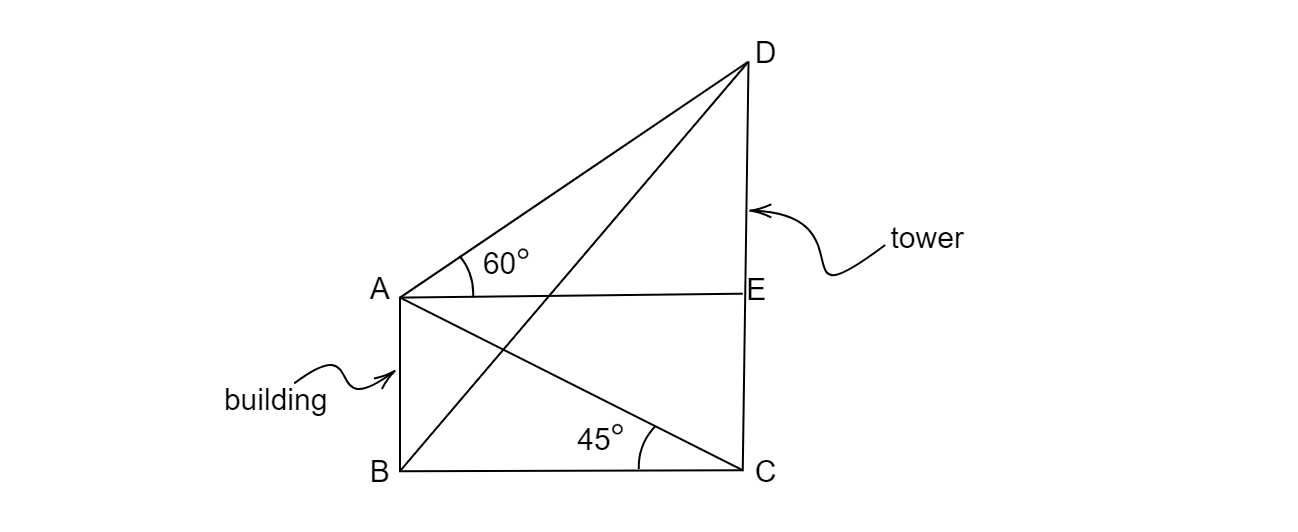# From the top of a 7 m high building, the angle of the elevation of the top of a tower is $60^{o}$ and the angle of the depression of the foot of the tower is $30^{o}$. Find the height of the tower.

Given: Height of the building$=7$ m, angle of the elevation off the top of the tower$=60^{o}$ and the angle of depression of the foot of the tower$=30^{o}$
To do: To find out the height of the tower.
Solution:
Let AB is the building and CD is towerAs given
$\angle EAD=60^{o}$

$\because AE\parallel BC$

$\Rightarrow \angle ACB=\angle EAC=45^{o}$

In $\vartriangle ABC$, $tan45^{o} =\frac{AB}{BC} =\frac{7}{BC} =1$          $( \because tan45^{o} =1)$

$BC=7$

$BC=AE=7$

Now in $\vartriangle AED$

$tan60^{o} =\sqrt{3} =\frac{DE}{AE} =\frac{DE}{7}$

$\Rightarrow DE=7\sqrt{3}$

We know that the height of the tower is $CD=CE+ED=7+7\sqrt{3} =7\left( 1+\sqrt{3}\right)$

$=19.13$ m.

$\therefore$ Height of the tower is 19.13 m

Updated on: 10-Oct-2022

75 Views